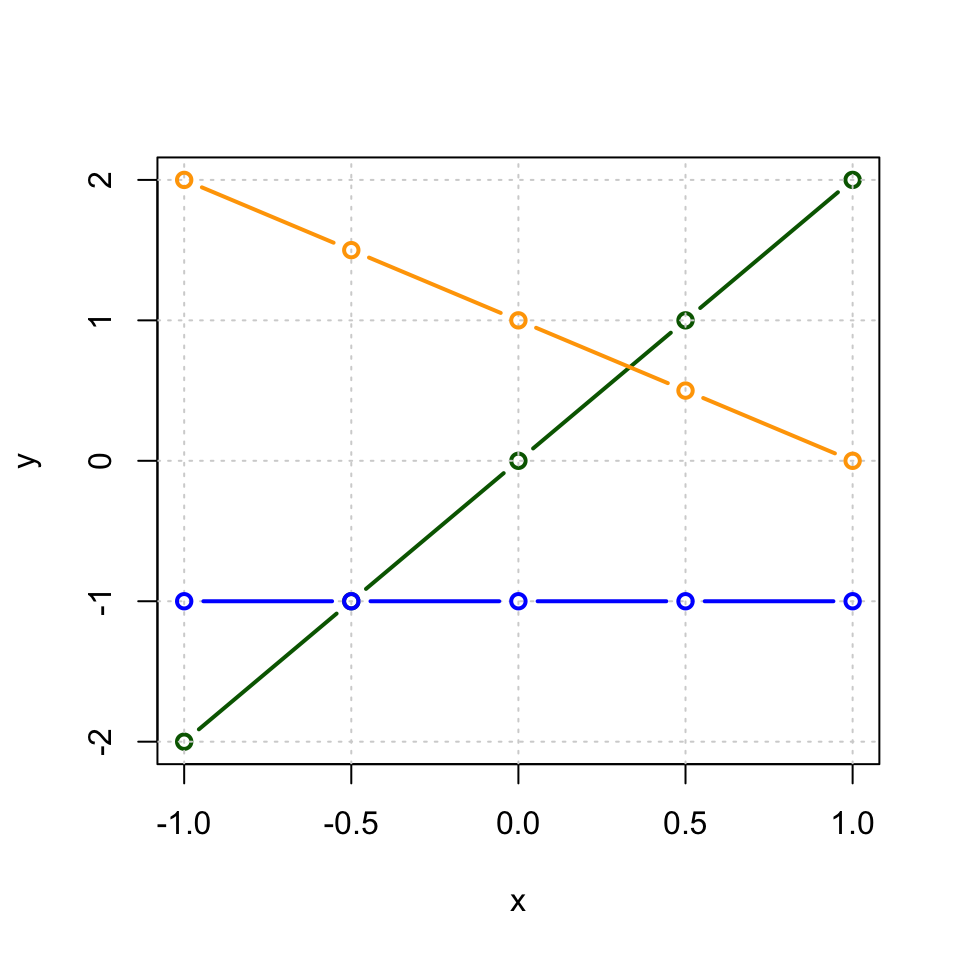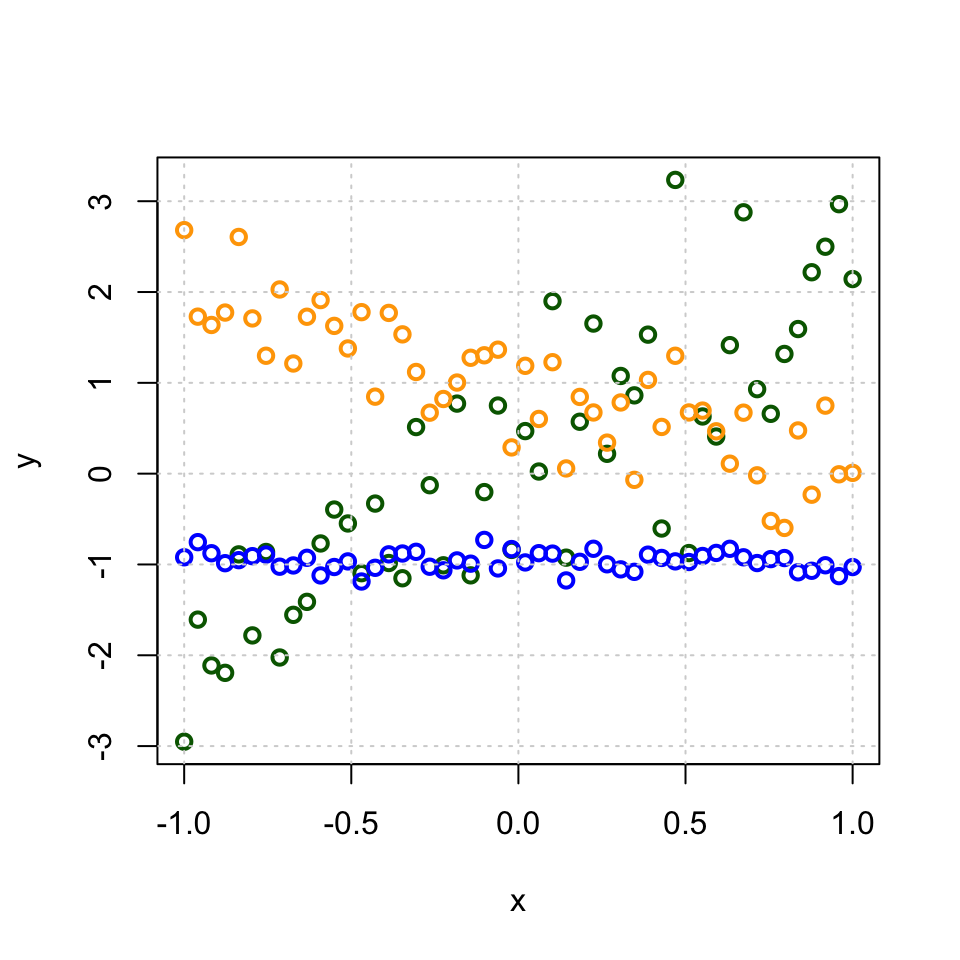In this lecture, we study relationship between two or more variables. First, we establish how to quantify the strength of the linear relationship between continuous variables and then we learn how to use the relationship to predict value of an unknown variable given the values of observed variables.

What is a linear relationship?

Mathematically, two variables $$X$$ and $$Y$$ are linearly related if there are some numbers $$a$$ and $$b$$ such that $$Y = a + b \cdot X$$. Here, $$b$$ is the coefficient that links the changes in $$X$$ to changes in $$Y$$: A change of one unit in variable $$X$$ always corresponds to a change of $$b$$ units in variable $$Y$$. Additionally, $$a$$ is the value that allows a shift between the ranges of $$X$$ and $$Y$$.

Let’s plot three linear relationships with parameters $$a = 0, b= 2$$ in green $$a=1, b=-1$$ in orange and $$a= -1, b=0$$ in blue. Let’s use 5 points to demonstrate these two lines when x-coordinate is between -1 and 1.

n = 5
x = seq(-1, 1, length = n)
y = 0 + 2*x
plot(x, y, t = "b", col = "darkgreen", lwd = 2) #t="b" uses "b"oth lines and points
grid() #make a grid on background
y = 1 + (-1)*x
lines(x, y, t = "b", col = "orange", lwd = 2) # add line to the existing plot
y = -1 + 0*x
lines(x, y, t = "b", col = "blue", lwd = 2) # add line to the existing plotWe see that depending on the sign of $$b$$, the line is either increasing ($$b=2$$, green), decreasing ($$b=-1$$, orange), or flat ($$b=0$$, blue). We call $$b$$ as slope (kulmakerroin) and $$a$$ as intercept (vakiotermi) of the linear relationship.

Example. Friedewald’s formula is an example of a linear relationship. It tells how to estimate LDL cholesterol values from total cholesterol, HDL cholesterol and triglycerides (when measured in mmol/l): $\text{LDL}\approx \text{TotalC} - \text{HDL} - 0.45\cdot \text{TriGly}.$

In practice, we never observe perfect linear relationships between measurements. Rather we observe relationships that are linear to some degree, and that are further diluted by noise in the measurements. We can model such imperfect linear relationships by adding some Normally distributed random variation on top of a perfect linear relationships of the previous figure. Let’s add most noise to the green and least to the blue line. The amount of noise is determined by the standard deviation of the Normal variable that is added on top of the perfect linear relationship, where larger SD means that we are making a more noisy observation of the underlying line.

n = 50
x = seq(-1, 1, length = n)
y = 0 + 2*x + rnorm(n,0,0.8)
plot(x, y, t = "p", col = "darkgreen", lwd = 2) #t="b" uses "b"oth lineas and points
y = 1 + -1*x + rnorm(n,0,0.4)
lines(x, y, t = "p", col = "orange", lwd = 2) # add line to the existing plot
y = -1 + 0*x + rnorm(n,0,0.1)
lines(x, y, t = "p", col = "blue", lwd = 2) # add line to the existing plot
grid() #make a grid on backgroundIn this Figure, the quality of the linear model as an explanation of the relationship between X and Y varies between the three cases (blue best, green worst). Next we want to quantify such differences.

Correlation

We read in a data set of heights and weights of 199 individuals (88 males and 111 females). This dataset originates from http://vincentarelbundock.github.io/Rdatasets/doc/carData/Davis.html. You can download it from the course webpage. We will call it measures.

measures = read.table("Davis_height_weight.txt",as.is = T, header = T) #using 'T' as a shorthand for 'TRUE'
plot(measures$height, measures$weight, pch = 20, #pch = 20 means a solid round symbol